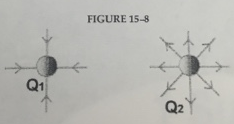# Problem: 1. The charge earned by one electron is e = -1.6×10-19 C. The number of electrons necessary to produce a charge of -1.0 C is A). 6.3×1018B) 1.6×109 C) 6.3×109 D) 1.6×109 E) none of the above 2. The force of attraction between a -40.0 μC and + 108 μC charge is 4.00 N. What is the separation between these two charges? A) 1.13 m B)2.10 m C) 3.12 m D) 2.49 m E) 3.67 m 3. An atomic nucleus has a charge of +40e An electron is 10-9 m from the nucleus. What is the force on the electron? A) 2.9 nN B) 9.2 nN C) 3.7 nN D) 1000 C E) 6.8 nN 4. A force of 10 N acts on a charge of 5.0 μC when it is placed in a uniform electric field. What is the magnitude of this electric field? A) 1.0 MN/C B) 50MN/C C) 0.50 MN/C D) 1000 MN/C E) 2.0 MN/C 5. Fig. 15-8 shows electric field lines near two electric point charges. If Q1 = -1 μC, what is the value of Q2? A) -1 μC B) 0 C) +1 μC D) -2 μC E) 2 μC

###### FREE Expert Solution

1.

The charge of an electron is given by:

1e = - 1.6 × 10-19 C

94% (286 ratings)###### Problem Details

1. The charge earned by one electron is e = -1.6×10-19 C. The number of electrons necessary to produce a charge of -1.0 C is

A). 6.3×1018

B) 1.6×109

C) 6.3×109

D) 1.6×109

E) none of the above

2. The force of attraction between a -40.0 μC and + 108 μC charge is 4.00 N. What is the separation between these two charges?

A) 1.13 m

B)2.10 m

C) 3.12 m

D) 2.49 m

E) 3.67 m

3. An atomic nucleus has a charge of +40e An electron is 10-9 m from the nucleus. What is the force on the electron?

A) 2.9 nN

B) 9.2 nN

C) 3.7 nN

D) 1000 C

E) 6.8 nN

4. A force of 10 N acts on a charge of 5.0 μC when it is placed in a uniform electric field. What is the magnitude of this electric field?

A) 1.0 MN/C

B) 50MN/C

C) 0.50 MN/C

D) 1000 MN/C

E) 2.0 MN/C5. Fig. 15-8 shows electric field lines near two electric point charges. If Q1 = -1 μC, what is the value of Q2?

A) -1 μC

B) 0

C) +1 μC

D) -2 μC

E) 2 μC